# C Tutorial | C Programming Tutorials | C Programming Notes | All In One C - Tutorials by CodeTextPro

In this C tutorials, we will learn more about data type in c, what is data type in c, double data type in c, enumerated data type in c, abstract data type in c, long data type in c, void data type in c, data type size in c, short data type in c, c programming notes, c languages notes, c notes, switch statement in c, nested if-else statement, if-else statement, if statement, c decision making, floating-point data type in c, primary data type in c, c variable, keyword in c languages, etc.
This is an all in one C tutorials Notes provided by CodeTextPro.com
C tutorials | C Programming Tutorials | C programming Notes

A programming language is proposed to help programmer to process certain kinds of data and to provide useful output. The task of data processing is accomplished by executing series of commands called program. A program usually contains different types of data types (integer, float, character etc.) and need to store the values being used in the program. C language is rich of data types. A C programmer has to employ proper data type as per his requirements.

C has different data types for different types of data and can be broadly classified as:
1. Primary Data Types

2. Secondary Data Types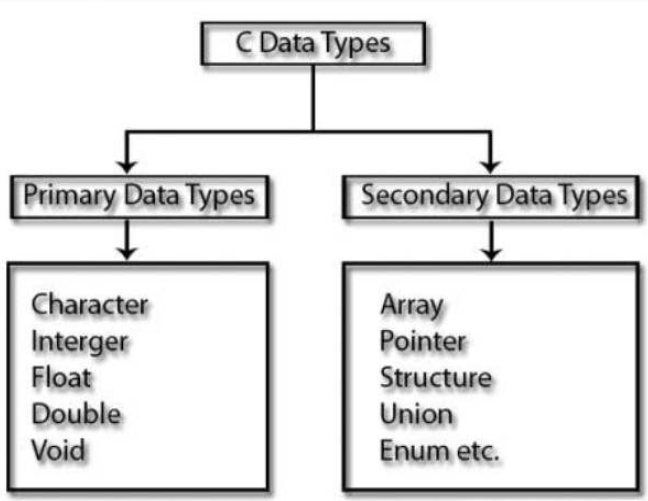C data type

Primary Data Types:
Integer Data Types:
Integers are whole numbers with a range of values, range of values are machine dependent. Generally an integer
occupies 2 bytes memory space and its value range limited to -32768 to +32767 (that is, -215 to +215-1). A signed
integer use one bit for storing sign and rest 15 bits for number.

To control the range of numbers and storage space, C has three classes of integer storage namely short int, int and
long int. All three data types have signed and unsigned forms. A short int requires half the amount of storage than
normal integer. Unlike signed integer, unsigned integers are always positive and use all the bits for the magnitude of
the number. Therefore, the range of an unsigned integer will be from 0 to 65535. The long integers are used to declare a longer range of values and it occupies 4 bytes of storage space.

Syntax:
int <variable name>;
int num1;
short int num2;
long int num3;

Example:  5, 6, 100, 2500.

Integer Data Type Memory Allocation: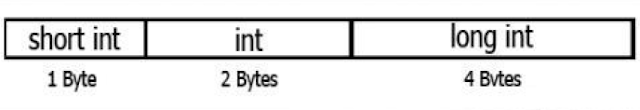Primary Data Type / Integer Data Type

Floating Point Data Types:
The float data type is used to store fractional numbers (real numbers) with 6 digits of precision. Floating point numbers are denoted by the keyword float. When the accuracy of the floating point number is insufficient, we can use the double to define the number. The double is same as float but withn longer precision and takes double space (8 bytes) than float. To extend the precision further we can use long double which occupies 10 bytes of memory space.

Syntax:
float <variable name>;
float num1;
double num2;
long double num3;

Example: 9.125, 3.1254.

Floating Point Data Type Memory Allocation:Floating Point Data Type

Character Data Type:
Character type variable can hold a single character and are declared by using the keyword char. As there are singed
and unsigned int (either short or long), in the same way there are signed and unsigned chars; both occupy 1 byte each, but having different ranges. Unsigned characters have values between 0 and 255, signed characters have values from –128 to 127.

Syntax:
char <variable name>;
char ch = ‘a’;

Example:   a, b, g, S, j.

Void Type:
The void type has no values therefore we cannot declare it as variable as we did in case of integer and float. The void

data type is usually used with function to specify its type.

MODIFIERS IN C LANGUAGE:

● The amount of memory space to be allocated for a variable is derived by modifiers.

● Modifiers are prefixed with basic data types to modify (either increase or decrease) the amount of storage space allocated to a variable.

● For example, storage space for int data type is 4 byte for 32 bit processor. We can increase the range by using long int which is 8 byte. We can decrease the range by using short int which is 2 byte.

● There are 5 modifiers available in C language. They are,

1. short
2. long
3. signed
4. unsigned

5. long long

Below table gives the detail about the storage size of each C basic data type in 16 bit processor. Please keep in mind that storage size and range for int and float datatype will vary depend on the CPU processor (8,16, 32 and 64 bit)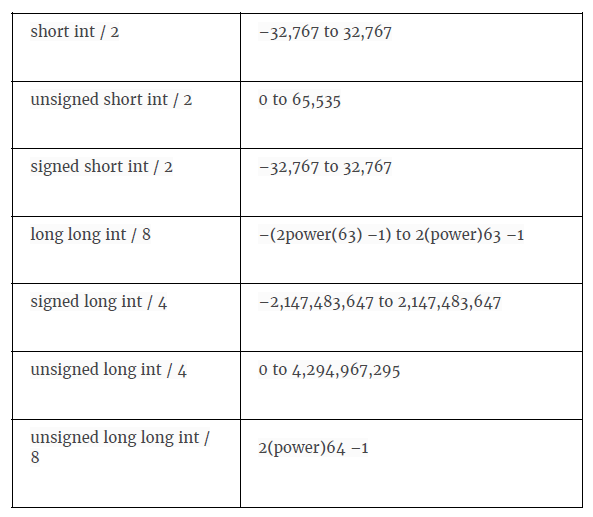MODIFIERS IN C LANGUAGE

ENUMERATION DATA TYPE IN C LANGUAGE:
● Enumeration data type consists of named integer constants as a list.
● It start with 0 (zero) by default and value is incremented by 1 for the sequential
identifiers in the list.
● Enum syntax in C:
● enum identifier [optional{ enumerator-list }];

● Enum example in C:

enum month { Jan, Feb, Mar }; or

/* Jan, Feb and Mar variables will be assigned to 0, 1 and 2 respectively by default */

enum month { Jan = 1, Feb, Mar };

/* Feb and Mar variables will be assigned to 2 and 3 respectively by default */

enum month { Jan = 20, Feb, Mar };

/* Jan is assigned to 20. Feb and Mar variables will be assigned to 21 and 22 respectively by default */

VOID DATA TYPE IN C LANGUAGE:
● Void is an empty data type that has no value.
● This can be used in functions and pointers.
● Please visit “ C – Function ” to pic to know how to use void data type in function with simple call by value and call by reference example programs.

KEYWORDS IN C LANGUAGE:

● Keywords are pre-defined words in a C compiler.
● Each keyword is meant to perform a specific function in a C program.
● Since keywords are referred names for compiler, they can’t be used as variable name.

C language supports 32 keywords which are given below. Click on each keywords below for detail description and example programs.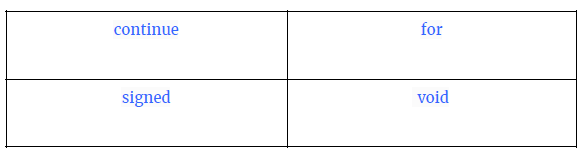KEYWORDS IN C LANGUAGEKEYWORDS IN C LANGUAGE

C – Variable
● C variable is a named location in a memory where a program can manipulate the data. This location is used to hold the value of the variable.
● The value of the C variable may get change in the program.
● C variable might be belonging to any of the data type like int, float, char etc.

RULES FOR NAMING C VARIABLE:
1. Variable name must begin with letter or underscore.
2. Variables are case sensitive
3. They can be constructed with digits, letters.
4. No special symbols are allowed other than underscore.
5. sum, height, _value are some examples for variable name

DECLARING & INITIALIZING C VARIABLE:
● Variables should be declared in the C program before to use.
● Memory space is not allocated for a variable while declaration. It happens only on variable definition.
● Variable initialization means assigning a value to the variable.DECLARING & INITIALIZING C VARIABLE

THERE ARE THREE TYPES OF VARIABLES IN C PROGRAM THEY ARE,
1. Local variable
2. Global variable
3. Environment variable

DIFFERENCE BETWEEN VARIABLE DECLARATION & DEFINITION IN C: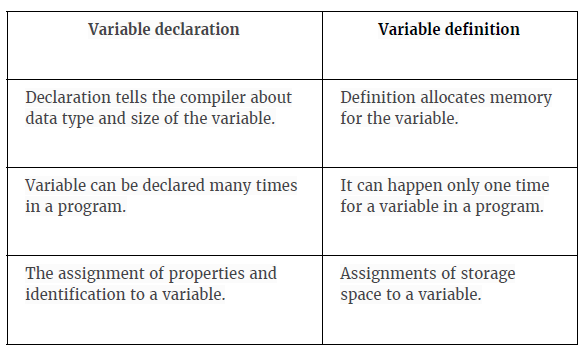DIFFERENCE BETWEEN VARIABLE DECLARATION and DEFINITION

C – Operators and Expressions
● The symbols which are used to perform logical and mathematical operations in a C program are called C operators.
● These C operators join individual constants and variables to form expressions.
● Operators, functions, constants and variables are combined together to form expressions.
● Consider the expression A + B * 5. where, +, * are operators, A, B are variables, 5 is constant and A + B * 5 is an expression.

TYPES OF C OPERATORS:

C language offers many types of operators. They are,

1. Arithmetic operators
2. Assignment operators
3. Relational operators
4. Logical operators
5. Bitwise operators
6. Conditional operators (ternary operators)
7. Increment/decrement operators
8. Special operators

ASSIGNMENT OPERATORS IN C:
● In C programs, values for the variables are assigned using assignment operators.

● For example, if the value “10” is to be assigned for the variable “sum”, it can be assigned as “sum = 10;”

● There are 2 categories of assignment operators in C language. They are,

● 1. Simple assignment operator ( Example: = )

● 2. Compound assignment operators ( Example: +=, -=, *=, /=, %=, &=, ^= )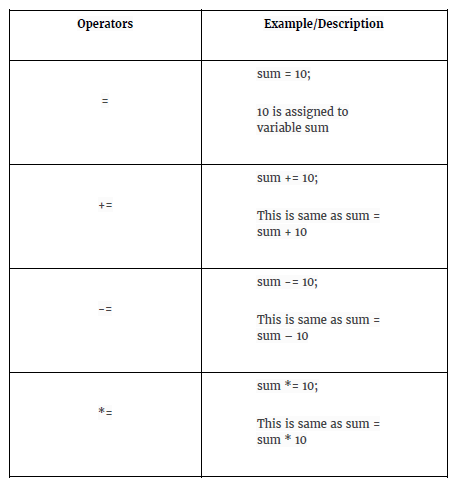ASSIGNMENT OPERATORS IN CASSIGNMENT OPERATORS IN C

LOGICAL OPERATORS IN C:

● These operators are used to perform logical operations on the given expressions.

● There are 3 logical operators in C language. They are, logical AND (&&), logical OR (||) and logical NOT (!).C Logical operators

BIT WISE OPERATORS IN C:

● These operators are used to perform bit operations. Decimal values are converted into binary values which are the sequence of bits and bitwise operators work on these bits.

● Bitwise operators in C language are & (bitwise AND), | (bitwise OR), ~ (bitwise OR), ^ (XOR), << (left shift) and >> (right shift).

TRUTH TABLE FOR BITWISE OPERATION & BIT WISE OPERATORS:TRUTH TABLE FOR BITWISE OPERATION & BIT WISE OPERATORS

BELOW ARE THE BIT-WISE OPERATORS AND THEIR NAME IN C LANGUAGE.

1. & – Bitwise AND
2. | – Bitwise OR
3. ~ – Bitwise NOT
4. ^ – XOR
5. << – Left Shift
6. >> – Right Shift

Consider x=40 and y=80. Binary form of these values are given below.

x = 00101000

y= 01010000

All bitwise operations for x and y are given below.

1. x&y = 00000000 (binary) = 0 (decimal)

2. x|y = 01111000 (binary) = 120 (decimal)

3. ~x = 11111111111111111111111111
11111111111111111111111111111111010111 = -41 (decimal)

4. x^y = 01111000 (binary) = 120 (decimal)

5. x << 1 = 01010000 (binary) = 80 (decimal)

6. x >> 1 = 00010100 (binary) = 20 (decimal)

Note:

Bitwise NOT: Value of 40 in binary is 00000000000000000000000000000000
00000000000000000010100000000000. So, all 0’s are converted into 1’s in bitwise NOT operation.

Bit wise left shift and right shift : In left shift operation “x << 1 “, 1 means that the bits will be left shifted by one place. If we use it as “x << 2 “, then, it means that the bits will be left shifted by 2 places.

C - Decision Making

Decision making is about deciding the order of execution of statements based on certain conditions or repeat a group of statements until certain specified conditions are met. C language handles decision-making by supporting the following statements,

● if statement
● switch statement
● conditional operator statement
● goto statement

Decision making with if statement

The if statement may be implemented in different forms depending on the complexity of conditions to be tested. The different forms are,

1. Simple if statement
2. If....else statement
3. Nested if....else statement
4. else if statement

Simple if statement

The general form of a simple if statement is ,
if( expression )
{
statement inside;
}
statement outside;

If the expression is true, then 'statement-inside' it will be executed, otherwise 'statement-inside' is skipped and only 'statement-outside' is executed.

Example :
#include <stdio.h>
int main( )
{
int x,y;
x=15;
y=13;
if (x > y )
{
printf("x is greater than y");
}
return 0;
}

Output
x is greater than y

if...else statement

The general form of a simple if...else statement is,

if( expression )
{
statement block1;
}
else
{
statement block2;
}

If the 'expression' is true, the 'statement-block1' is executed, else 'statement-block1' is skipped and 'statement-block2' is executed.

Example :

#include <stdio.h>
int main( )
{
int x,y;
x=15;
y=18;
if (x > y )
{
printf("x is greater than y");
}
else
{
printf("y is greater than x");
}
return 0;
}

Output

y is greater than x

Nested if....else statement

The general form of a nested if...else statement is,

if( expression )
{
if( expression1 )
{
statement block1;
}
else
{
statement block2;
}
}
else
{
statement block3;
}

if 'expression' is false the 'statement-block3' will be executed, otherwise, it continues to perform the test for 'expression 1' . If the 'expression 1' is true the 'statement-block1' is executed otherwise 'statement-block2' is executed.

Example :

#include <stdio.h>
int main( )
{
int a,b,c;
clrscr();
printf("enter 3 number");
scanf("%d%d%d",&a,&b,&c);
if(a>b)
{
if( a > c)
{
printf("a is greatest");
}
else
{
printf("c is greatest");
}
}
else
{
if( b> c)
{
printf("b is greatest");
}
else
{
printf("c is greatest");
}
}
return 0;

}

The general form of else-if ladder is,

if(expression1)
{
statement block1;
}
else if(expression2)
{
statement block2;
}
else if(expression3 )
{
statement block3;
}
else

default statement;

The expression is tested from the top(of the ladder) downwards. As soon as the true condition is found, the statement associated with it is executed.

Example :

#include <stdio.h>
int main( )
{
int a;
printf("enter a number");
scanf("%d",&a);
if( a%5==0 && a%8==0)
{
printf("divisible by both 5 and 8");
}
else if( a%8==0 )
{
printf("divisible by 8");
}
else if(a%5==0)
{
printf("divisible by 5");
}
else
{
printf("divisible by none");
}

}

Switch statement

Switch statement is used to solve multiple option type problems for menu like program, where one value is associated with each option. The expression in switch case evaluates to return an integral value, which is then compared to the values in different cases, where it matches that block of code is executed, if there is no match, then default block is executed. The general form of switch statement is,

switch(expression)
{
case value-1:
block-1;
break;

case value-2:
block-2;
break;

case value-3:
block-3;
break;

case value-4:
block-4;
break;

default:
default-block;
break;

}

Points to Remember

1. We don't use those expressions to evaluate switch case, which may return floating point values or strings.

2. It isn't necessary to use break after each block, but if you do not use it, all the consecutive block of codes will get executed after the matching block.

3. int i = 1;
switch(i)
{
case 1:
printf("A"); // No break

case 2:
printf("B"); // No break

case 3:
printf("C");
break;

}

4. Output: A B C

5. The output was supposed to be only A because only the first case matches, but as there is no break statement after the block, the next blocks are executed, until the cursor encounters a break.

6. The default case can be placed anywhere in the switch case. Even if we don't include the default case switch statement works.

Example of Switch Statement

#include<stdio.h>
int main( )
{
int a,b,c,choice;
while(choice!=3)
{
printf("\n 1. Press 1 for addition");
printf("\n 2. Press 2 for subtraction");
scanf("%d",&choice);
switch(choice)
{
case 1:
printf("Enter 2 numbers");
scanf("%d%d",&a,&b);
c=a+b;
printf("%d",c);
break;
case 2:
printf("Enter 2 numbers");
scanf("%d%d",&a,&b);
c=a-b;
printf("%d",c);
break;
default:
printf("you have passed a wrong key") ;
printf("\n press any key to continue") ;
}
}
return 0;

}